# The non-ideal behavior of gases

in StemSocial2 months ago
Greetings dear friends of the Hive scientific community.

We all know very well the general behavior of gases, i.e., we know that it is a state of matter whose main characteristic is the little interaction between its molecules, so they do not form molecular bonds, which allows them to separate and occupy the entire volume of the container that contains them.under normal conditions, most gases behave in a similar way. Image credit: piqsels.com.

And because gases behave similarly over a wide range of conditions, there are mathematical models that allow us to estimate ideally how a gas behaves, establishing equations that account for the relationship between pressure, temperature and volume, and giving rise to the ideal gas law expressed by Clapeyron in 1934.

I am sure that many of us have heard enough of this ideal gas law, and that by now we have at least a notion of how to use the well-known relationship PV = nRT.

## But sometimes life is not ideal

This equation is a simplification of reality, these postulates ignore the volume occupied by the molecules of a gas, since they consider that it is very small compared to the volume they occupy, and also ignore all interactions between molecules, assuming that they do not attract or repel each other. So, what happens if we want to study a gas that behaves differently? That is, non-ideal.

Let us consider in this article the properties of gases known to be real, and why they differ from the estimates made with the ideal gas equation.

## What is a real gas?

As we have mentioned before, a real gas is one that does not conform to idealized behavior, that is, one that exhibits properties that cannot be described by the well-known ideal gas law enunciated by Clapeyron, and which is expressed as follows: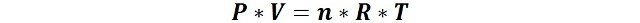Where, n are the moles of gas, P is the pressure, V the volume, R the gas constant and T the temperature.

This law is based on assumptions such as the consideration of gas molecules as point particles, without volume, and that there is no attractive force between them.

But in real gases, under certain conditions of pressure and temperature, their intermolecular interactions increase, and in reduced volumes the volume ratio of the molecules to the total volume increases. That is why, at certain low temperatures and at high pressure, real gases undergo a phase transition, such as liquefaction, something that the equation of an ideal gas does not allow.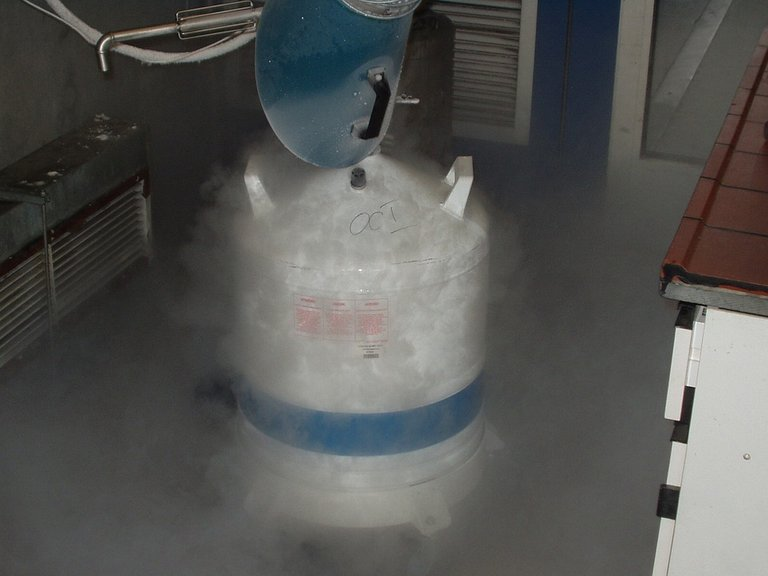If nitrogen behaved ideally, there would be no such thing as cryogenic liquid. Image credit: pixabay.com.

Real gases can be diatomic molecules such as oxygen (O2) and nitrogen (N2), or monoatomic molecules such as helium (He), even heavier gases such as carbon dioxide (CO2) and methane (CH4).

## Characteristics of real gases

• Molecules exert attractive or repulsive forces on each other
Between gases there are attractive forces known as Van der Waals forces, which tend to bind them together limiting their mobility. And there are also repulsive forces of the dipole-dipole type, known as London forces. Especially at high pressures, there is more interaction between the molecules.

• Molecules have volume
In the idealized model the volume occupied by the molecules is neglected, considering it to be too small in contrast to the total volume occupied with the gas. And it works quite well over a wide range of conditions, but, the volume of the molecules starts to become important when the gas is subjected to sufficient pressure to reduce its volume.

## Compressibility factor, a measure of ideal behavior

When considering the above characteristics, significant deviations from the ideal model are obtained. For an ideal gas, a plot of PV/nRT versus P gives a horizontal line with an intercept of 1 on the PV/nRT axis. Real gases, however, show significant deviations from the expected behavior for an ideal gas, especially at high pressures.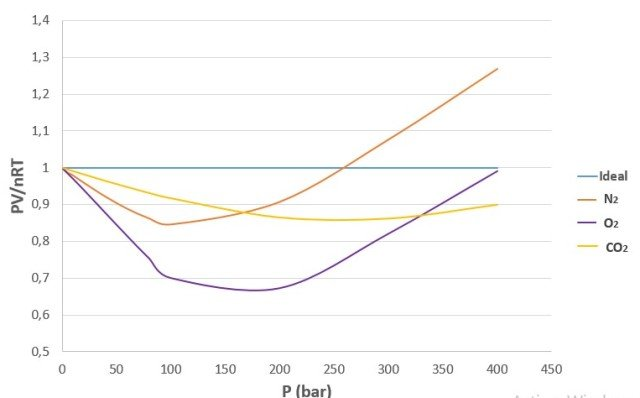Graph of PV/nRT versus P at 200 K for several common gases. Source: @emiliomoron, prepared with data taken from Perry, R. (1992).

The PV/nRT factor is also known as compressibility factor z, and is a ratio for which, in ideal gases it has a value equal to 1 regardless of conditions, whereas in the example gases, such as N2, O2 and CO2, it is variable and changes with gas pressure and temperature.

That is why one way to know the precision with which the ideal gas law describes our system under study, we can take n moles of our gas at a certain temperature and measure the molar volume, Vm, that it occupies at a certain pressure, and we can calculate the molar volume of the ideal gas at the same temperature and pressure, and then find the ratio between the two volumes.## Van der Waals equation. When Intermolecular Forces Matter

Gases can conform to the ideal gas equation (PV = nRT) under specific conditions; but not under all conditions, especially low temperatures and high pressures, producing the need to correct the law established for these cases.

In an attempt to explain why real gases did not obey the ideal gas law (PV = nRT), the Dutch physicist J. D. van der Waals (1837-1923) considered the effects of attractive forces between molecules, something that by the time the conception of molecules as we know them was still the subject of intense debate. And in 1873 he arrived at his famous equation of state for gases and liquids,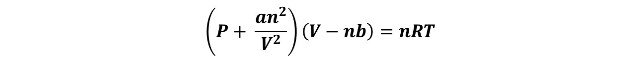In this equation the term a/V2 was added to the external (measured) pressure P to account for attractive intermolecular forces; at low pressures there is too little attractive interaction between molecules to diminish the impact of each molecule hitting the vessel wall, and the pressure is very close to that predicted by the ideal gas law, but at high pressures, the distance between molecules is relatively small, and the surrounding molecules tend to diminish the impact of a given molecule against the vessel wall, resulting in a lower pressure than predicted by the ideal gas law.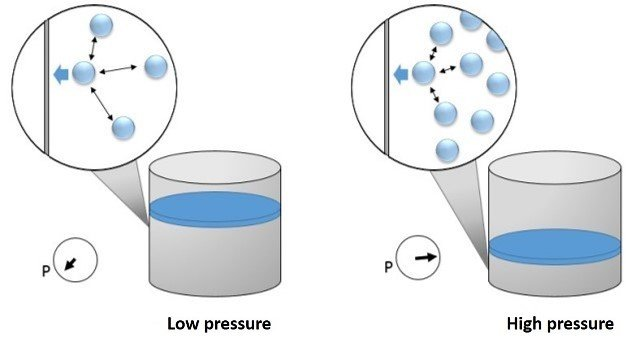Effect of intermolecular attractive forces on the pressure exerted by a gas on the vessel walls. Source: image by @emiliomoron.

This term "a" is an empirical constant that is specific to each gas and has as unit L2.atm.mol-2-.

The equation also includes the factor "b" subtracting to the volume, to account for the finite size of the molecules, that is, to include the volume occupied by the gas molecules, this factor is obtained empirically and has as unit: L.mol-1. For most gases we can find these constants in the literature.

The equation also includes the factor "b" subtracting to the volume, thus to account for the finite size of the molecules, i.e. to include the volume occupied by the gas molecules, this factor is obtained empirically and has as unit: L.mol-1. For some gases they are listed in the following table.

Table 1. Van der Waals constants for some gases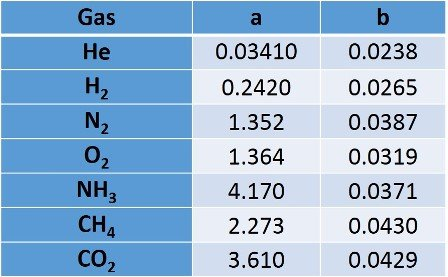## Application of the Van der Waals Equation

Let's say you are in charge of supervising the manufacture of gas cylinders, and the company wants to offer a 10 L cylinder containing 500 g of methane gas. If the containers you have available to manufacture them have a burst pressure of 70 atm at 25 °C, would it be safe to manufacture them? Let's use the ideal gas equation and Van der Waals equation to find out.

From these data we have the volume of the cylinder, mass of the compound, pressure and temperature.

Let us first determine the moles of gas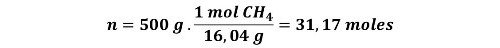A. Using the ideal gas equation at a temperature of 298 K (25 °C)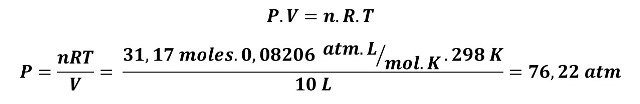If methane behaved as an ideal gas there would be a problem, the cylinders would not withstand the pressure of the gas.

B. Now let's use the Van der Waals equation with the a and b values from Table 1 for methane.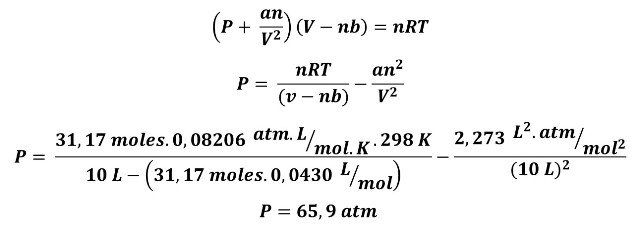This pressure is within the safety limits of the cylinder. Using the above equation would represent for the company to invest in an oversized material.

In summary, the ideal gas equation works well when the intermolecular attraction between the gas molecules can be considered negligible and the space occupied by the gas molecules is not an important part of the total volume of the container, usually this is valid at low pressures (about 1 bar) and the temperature is high. In other cases, when the pressure is high and/or the temperature is low, the ideal gas law leads to different results from those that can be observed experimentally. For these situations, we can use the van der Waals equation to take into account that gases do not always behave ideally.

## References

1. Wikipedia.com. Gas real
2. Jacob N.Israelachvili (2011). Intermolecular and Surface Forces (Third Edition). Academic Press
3. Saylor Academy. The Behavior of Real Gases
Sort:

Congratulations @emiliomoron! You have completed the following achievement on the Hive blockchain and have been rewarded with new badge(s) :You distributed more than 10000 upvotes.Your next target is to reach 11000 upvotes.

If you no longer want to receive notifications, reply to this comment with the word `STOP`

Check out the last post from @hivebuzz: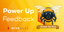Feedback from the March 1st Hive Power Up DayThanks for your contribution to the STEMsocial community. Feel free to join us on discord to get to know the rest of us!

Please consider supporting our funding proposal, approving our witness (@stem.witness) or delegating to the @stemsocial account (for some ROI).

Please consider using the STEMsocial app app and including @stemsocial as a beneficiary to get a stronger support.

Thanks for the support my friends!